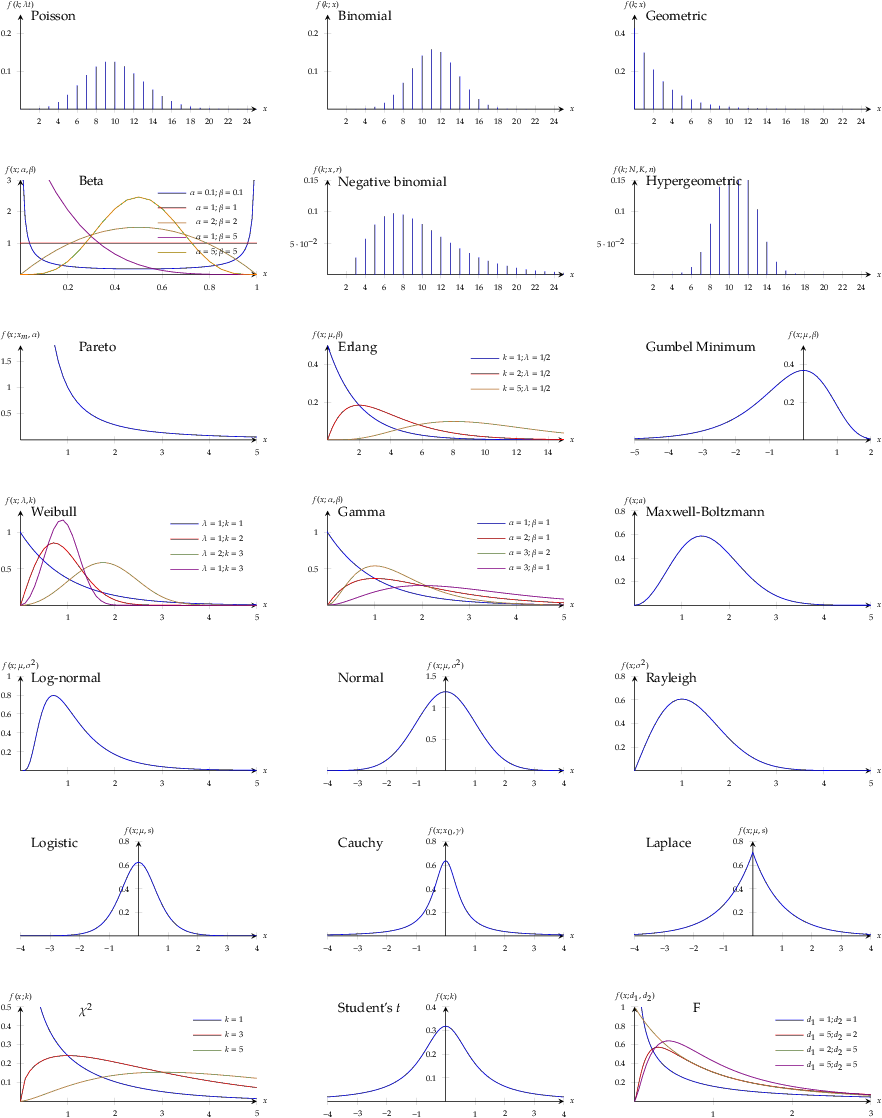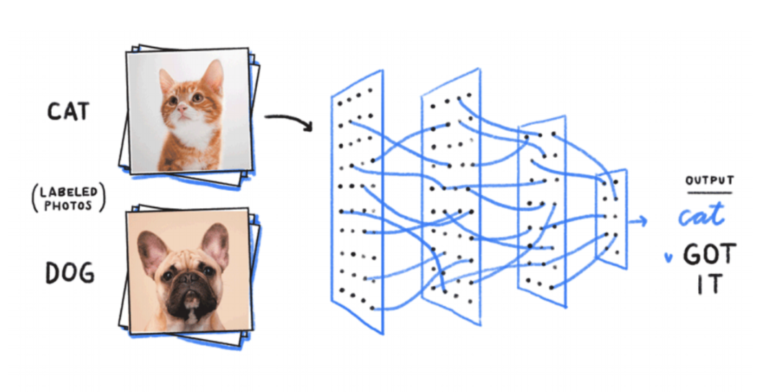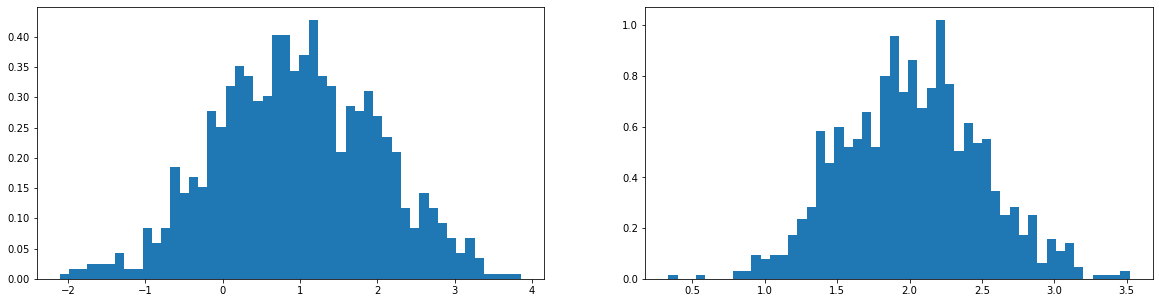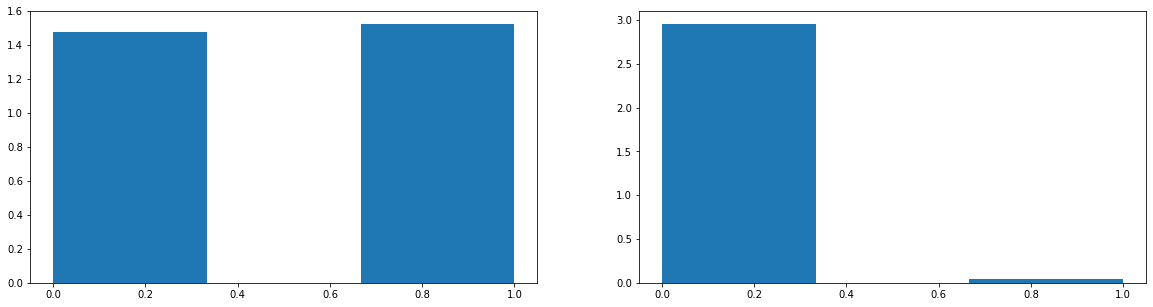### KL-Divergence, Relative Entropy in Deep Learning

Posted April 10, 2021 by Gowri Shankar  ‐  5 min read

This is the fourth post on Bayesian approach to ML models. Earlier we discussed uncertainty, entropy - measure of uncertainty, maximum likelihood estimation etc. In this post we are exploring KL-Divergence to calculate relative entropy between two distributions.

We shall see, how KL-Divergence works for Cats and Dogs classification problem.Image Credit: www.mentalfloss.com

Previous Posts in this Series

## Objective

Our objective is to get answers to the following questions

• What is KL Divergence?
• Why log likelihood?
• What is Likelihood ratio?
• Is expected values - Weighted average of instances of Random values?
• Is expected value of log likelihood ratio is KLD?
• What can KL-Divergence be used for?
• How are KL-Divergence and Cross Entropy related?
• Is KL-Divergence a distance measure?
• How are KL-Divergence and Log-Likelihood related?
• Is KLD asymmetric?
• What are forward and reverse KL?

## Introduction

KL-Divergence is a measure of how two distributions differ from each others. Some of very well known probability density distribution plotsImage Credit: ∫ntegrabℓε ∂iﬀerentiαℓs

Let us say we are building a deep neural network that classifies dogs and cats, for a dog picture - The probability of classifying a dog as dog by a perfect neural network is 1. i.e

$$\large p_{\theta}(dog) = 1, p_{\theta}(cat) = 0$$ where $\theta$ represents the reality.

Network Performance
However, our neural networks is governed by the estimated weights $\phi$. i.e for a decent neural network, outcome could be $$\large q_{\phi}(dog) = 0.8, q_{\phi}(cat) = 0.2$$ Meanwhile, if our network behaves adversarial to the expectations… we seek deeper explanations. i.e. $$\large q_{\phi}(dog) = 0.2, q_{\phi}(cat) = 0.8$$

Hence, we seek a clarity to declutter this uncertainity by measuring the probabilities relatively.

## KL-Divergence

In the above representations, we introduced two probabilities $p_{\theta}$ representing the distribution of the reality and $q_{\phi}$ representing the distribution of the estimator(DNN).

From a dataset $X$ with observations ${x_1, x_2, x_3, \cdots, x_n}$, the probability for a single observation($i$) can be written as below as a representation of two distributions, $$p_{\theta}(x_i), q_{\phi}(x_i)$$

A typical measure of difference is subtracting $p_{\theta}$ and $q_{\phi}$ but we are dealing with very small numbers which might result in rounding to zero. To avoid this, we compute log differences or loglikelihood. i.e instead of $$p_{\theta}(x_i) - q_{\phi}(x_i)$$ We take logarithmic difference $$log p_{\theta}(x_i) - log q_{\phi}(x_i)$$ $$i.e.$$ $$\large log \frac { p_{\theta}(x_i)}{q_{\phi}(x_i)} \tag{1. Log Likelihood Ratio}$$

We saw the behavior for a single observer $i$ but our interest is to understand the likelihood of the whole sample set. Which is what is the average likelihood or the tendency of the random variables

### KLD from Expected Value for Discrete Random Variables

Since every observation has a role in determining the probability, we have to give give importance to the ones with maximum likelihood. Hence a weighted average to all the observations are computed

$$\mathbb{E_{p\theta}}[h(X)] = \sum_{i=1}^\infty h(x_i)p_{\theta}(x_i) \tag{2. Expected Values}$$

Our goal is to find the average difference between the distributions $p_{\theta}$ and $q_{\phi}$, here expected values comes handy to calculate the average log likelihood ratio.

from eq.1 and eq.2

$$\sum_{i=1}^{\infty}p_{\theta}(x_i)log \frac {p_{\theta}(x_i)}{q_{\phi}(x_i)} \tag{3. Expected Log Likelihood Ratio}$$ $$i.e.$$ $$\large \mathbb{E_p}\left[log \frac {p_{\theta}(x)}{q_{\phi}(x)}\right ] = \sum_{i=1}^{\infty}p_{\theta}(x_i)\left[log \frac {p_{\theta}(x_i)}{q_{\phi}(x_i)}\right ] \tag{4. KL-Divergence}$$

### Continuous Random Varaibles

For continuous random variables $$D_{KL}(p_{\theta} || q_{\phi}) = \int_{\mathbb{R}} p_{\theta}(x)\left[log \frac {p_{\theta}(x)}{q_{\phi}(x)}\right ]dx \tag{5. Forward KLD}$$ $$and$$ $$D_{KL}(q_{\phi} || p_{\theta}) = \int_{\mathbb{R}} q_{\phi}(x)\left[log \frac {q_{\phi}(x)}{p_{\theta}(x)}\right ]dx \tag{6. Reverse KLD}$$

They are not symmetric, i.e. $$D_{KL}(p_{\theta} || q_{\phi}) \neq D_{KL}(q_{\phi} || p_{\theta})$$ Hence it is not distance measure but divergence.

Generally $p$ is the reference distribution and $q$ is approximation. Forward KLs are the cross-entropy losses widely used in machine learning.

### KL-Divergence as Loss Function

In this section let us explore how KL-Divergence is used as a loss function, from eqn.4

$$\large \sum_{i=1}^{\infty}p_{\theta}(x)log p_{\theta}(x) - \sum_{i=1}^{\infty}p_{\theta}(x)log q_{\phi}(x)$$

• The negative part is the cross-entropy $H(p_{\theta}, p_{\phi})$
• The positive part is the negative of entropy $H(p_{\theta}, p_{\theta})$, constant and derived from the dataset

Hence the loss function is $$\large - \sum_{i=1}^{\infty}p_{\theta}(x)log q_{\phi}(x)$$

## Cats and Dogs

We created 2 situations out of our neural network,

1. With a decent outcome and $$q_{\phi}(dog) = 0.8, q_{\phi}(cat) = 0.2$$
2. With adversarial outcome $$q_{\phi}(dog) = 0.2, q_{\phi}(cat) = 0.8$$

Let us calculate KL-Divergence between reality and estimationImage Credit: www.searchenginejournal.com

### Case 1: $q_{\phi}(dog) = 0.8, q_{\phi}(cat) = 0.2$

$$1.log_2 \left[\frac {1.0}{0.8}\right] + 0.log_2 \left[\frac {1}{0.2} \right]$$ $$i.e.$$ $$0.3219$$

Observation: KLD value is closer to zero

### Case 2: $q_{\phi}(dog) = 0.2, q_{\phi}(cat) = 0.8$

$$1.log_2 \left[\frac {1.0}{0.2}\right] + 0.log_2 \left[\frac {1}{0.8} \right]$$ $$i.e.$$ $$2.3219$$

Observation: KLD value is far from zero

### Case 3: A perfect model

$$1.log_2 \left[\frac {1.0}{1.0}\right] + 0.log_2 \left[\frac {1}{0.0} \right]$$ $$i.e.$$ $$0$$

Observation: KLD value is zero since it is a perfect model

$$\sum_{i=1}^{\infty}p_{\theta}(x_i)log \frac {p_{\theta}(x_i)}{q_{\phi}(x_i)} \geq 0$$

When two disributions are similar then the KL-Divergence value is close to zero and it increases as the intensity of differences of distributions increases

import tensorflow as tf
import tensorflow_probability as tfp
tfd = tfp.distributions
tfb = tfp.bijectors

import matplotlib.pyplot as plt


## Computing KL Divergence

In this section we shall explore how to use KL-Divergence API from Tensorflow Probabilities module

### Univariate Gausian Distributions

Let us compute KL divergence for two univariate normal distributions using Tensorflow Probabilities functions.

• Create $p$ and $q$ distributions, using $\mu = { 1, 2 }$ and $\sigma={ 1, 0.5 }$
• Compute the divergence using tensorflow distributions
𝜇_q = 1
𝜎_q = 1
𝜇_p = 2
𝜎_p = 0.5

distribution_q = tfd.Normal(loc=𝜇_q, scale=𝜎_q)
distribution_p = tfd.Normal(loc=𝜇_p, scale=𝜎_p)

n_samples = 1000
samples_q = distribution_q.sample(n_samples)
samples_p = distribution_p.sample(n_samples)

plt.figure(figsize=(20, 5))
plt.subplot(1, 2, 1)
plt.hist(samples_q, bins=50, density=True)
plt.subplot(1, 2, 2)
plt.hist(samples_p, bins=50, density=True)
plt.show()Observe the distribution mean and the spread in the above histograms. Further let us calculate the KL divergence

tfd.kl_divergence(distribution_q, distribution_p)

<tf.Tensor: shape=(), dtype=float32, numpy=2.8068528>


### Asymmetry

Note when we swap the distribution KLD value varies, hence it is not a measure of distance but divergence

tfd.kl_divergence(distribution_p, distribution_q)

<tf.Tensor: shape=(), dtype=float32, numpy=0.6371457>


### Fair Coin vs Unfair Coin

distribution_q = tfd.Categorical(probs=[0.5, 0.5])

distribution_p = tfd.Categorical(probs=[0.99, 0.01])

samples_q = distribution_q.sample(n_samples)
samples_p = distribution_p.sample(n_samples)

plt.figure(figsize=(20, 5))
plt.subplot(1, 2, 1)
plt.hist(samples_q, bins=3, density=True)
plt.subplot(1, 2, 2)
plt.hist(samples_p, bins=3, density=True)
plt.show()tfd.kl_divergence(distribution_q, distribution_p)

<tf.Tensor: shape=(), dtype=float32, numpy=1.6144631>


#### 2 Fair Coins

tfd.kl_divergence(distribution_q, distribution_q)

<tf.Tensor: shape=(), dtype=float32, numpy=0.0>


## Conclusion

In this post, we dived deep into KL-Divergence formula and how it is derived. Also learnt the significance of KL divergence in cross-entropy calculation. Further, we calculated KLD for various probability distributions understood the efficacy of the algorithm.